# 动态输出反馈系统不确定项容忍区间分析Tolerance Interval Analysis of System Uncertainty for Dynamic Output Feedback

DOI: 10.12677/DSC.2018.72011, PDF, HTML, XML, 下载: 695  浏览: 2,062  科研立项经费支持

Abstract: Considering linear time-invariable systems, based on the problem of pole assignment for the disk region of dynamic output feedback, we gave the concept of tolerance interval of single deviation model with uncertain parameters for control system. Through the analysis of the influence on the performance of the system by uncertain parameters fluctuation of linear time-invariable systems, we gave the algorithm of uncertain parameters tolerance interval in disk region by single deviation model of the system. Furthermore, by comparing the size of tolerance intervals for various uncertain parameters, we determine the level of influence on the performance of systems. The system designer can design a high quality control system by referring to the level of its influence. Finally, a vertical wing of an aircraft example is given to illustrate scientificalness and effectiveness of the conclusion.

1. 引言

2. 问题描述

$\left\{\begin{array}{l}\stackrel{˙}{x}\left(t\right)=Ax\left(t\right)+Bu\left(t\right)\\ y\left(t\right)=Cx\left(t\right)\end{array}$ (1)

$\left\{\begin{array}{l}\stackrel{˙}{\stackrel{˜}{x}}\left(t\right)={A}_{0}\stackrel{¯}{x}\left(t\right)+{B}_{0}y\\ u\left(t\right)={C}_{0}\stackrel{¯}{x}\left(t\right)+{D}_{0}y\end{array}$ (2)

$\left\{\begin{array}{l}\stackrel{˙}{\stackrel{˜}{x}}\left(t\right)={A}_{c}\stackrel{˜}{x}\left(t\right)\\ y\left(t\right)={C}_{c}\stackrel{˜}{x}\left(t\right)\end{array}$ (3)

$\left(\begin{array}{cc}-rX& qX+AX\\ qX+X{A}^{T}& -rX\end{array}\right)<0$ (4)

$\left[\begin{array}{cccc}-rX& -rI& XA+\stackrel{¯}{B}C+qX& \stackrel{¯}{A}+qI\\ \ast & -rY& A+qI& AY+B\stackrel{¯}{C}+qY\\ \ast & \ast & -rX& -rI\\ \ast & \ast & \ast & -rY\end{array}\right]<0$ (5)

$\left[\begin{array}{cc}X& I\\ I& Y\end{array}\right]>0$ (6)

$\begin{array}{l}{A}_{0}={M}^{-1}\left(\stackrel{¯}{A}-XAY-M{B}_{0}CY-XB{C}_{0}{N}^{\text{T}}\right){N}^{-\text{T}}\\ {B}_{0}={M}^{-1}\stackrel{¯}{B}\\ {C}_{0}=\stackrel{¯}{C}{N}^{-\text{T}}\end{array}$ (7)

$\left\{\begin{array}{l}\stackrel{˙}{\stackrel{˜}{x}}\left(t\right)={A}_{m}\stackrel{˜}{x}\left(t\right)\\ y\left(t\right)={C}_{c}\stackrel{˜}{x}\left(t\right)\end{array}$ (8)

1) $eig\left(A\right)$ 为矩阵A的特征值；

2) ${\lambda }_{ij}$ 为不确定因素第ij项波动时闭环系统的特征值；

3) $\mathrm{Re}\left[{\lambda }_{ij}\right]$${\lambda }_{ij}$ 的实部值；

4) $\mathrm{Im}\left({\lambda }_{ij}\right)$${\lambda }_{ij}$ 的虚部值

3. 主要结果

1) 必要性

${A}_{m}=\left[\begin{array}{cc}A+\Delta A+B{D}_{0}C& B{C}_{0}\\ {B}_{0}C& {A}_{0}\end{array}\right]$

2) 充分性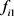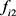为方程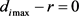的解。令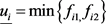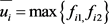，当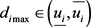时，线性系统的所有极点均在圆盘内。若，系统的部分或全部极点已经脱离圆盘，所以区间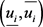为圆盘区域极点配置问题中线性系统不确定参数第 项因素波动的容忍区间。定理由此得以证明。

4. 仿真算例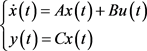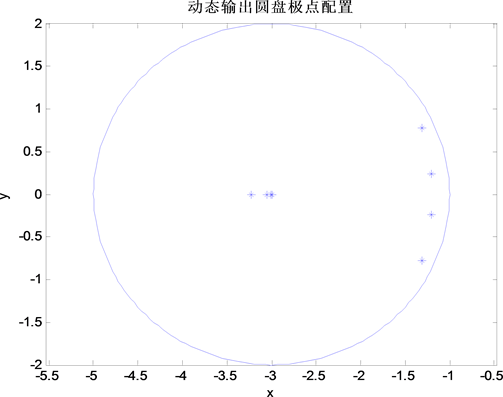Figure 1. Pole distribution graph of pole disk region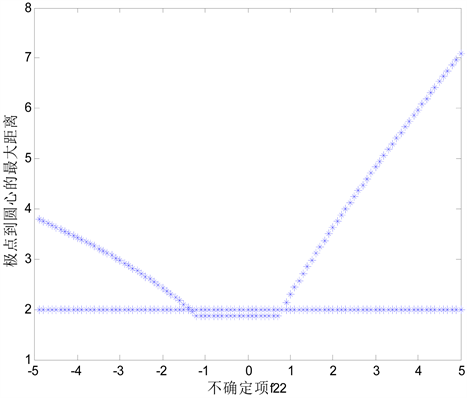Figure 2. Tolerance interval of parameter f22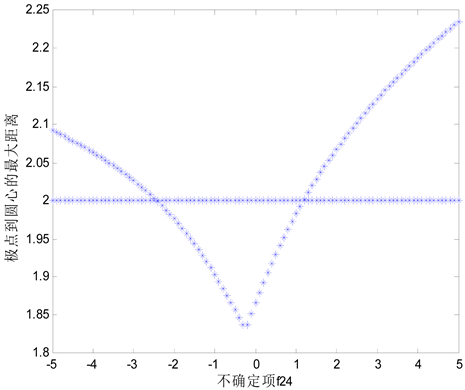Figure 3. Tolerance interval of parameter f24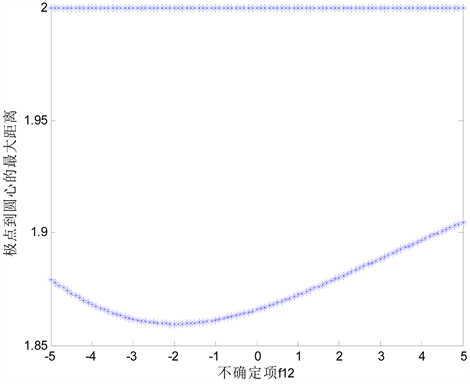Figure 4. Tolerance interval of parameter f12

5. 结论

  辛业春, 李国庆, 王朝斌, 吴学光. 基于状态反馈解耦控制的MMC-HVDC系统控制策略研究[J]. 电测与仪表, 2015, 52(20): 65-70.  滕青芳, 范多旺. 不确定系统的鲁棒状态反馈区域极点配置[J]. 计算技术与自动化, 2006(1): 8-10.  王吉华, 魏民祥, 杜言利, 李玉芳. 基于全状态观测器状态反馈的多轴转向极点配置[J]. 中国机械工程, 2013, 24(9): 1246-1251+1257.  王福忠, 闫浩. 基于动态输出反馈的时滞T-S模糊系统无源可靠控制[J]. 沈阳建筑大学学报(自然科学版), 2016, 32(4): 760-768.  王福忠, 沙漠. 奇异系统H_∞动态输出可靠控制[J]. 沈阳建筑大学学报(自然科学版), 2009, 25(5): 998-1002.  Azuma, T., Naito, H., Sagara, S., Fujita, M. and Uchida, K. (2003) Memory Observer-Based Output Feedback Control for Linear Time-Delay Systems. IFAC Proceedings Volumes, 36.  房志铭, 姚波, 王福忠. 基于区域极点配置的静态输出反馈可靠控制[J]. 宿州学院学报, 2017, 32(7): 100-105.  朱纪洪, 郭治. 不确定系统的动态输出反馈圆形区域极点配置[J]. 控制理论与应用, 1996(4): 489-494.  刘子平, 王福忠. 具有圆盘极点约束的H_∞性能指标容忍区间分析[J]. 数字技术与应用, 2011(11): 171-172.  王福忠, 辛健. 基于极点配置执行器增益偏差容忍区间的分析[J]. 控制工程, 2012, 19(5): 740-742.  于洁, 王福忠, 姚波, 王维宏. 梯形区域下执行器增益偏差容忍区间的分析[J]. 沈阳工程学院学报(自然科学版), 2015, 11(2): 171-175+188.  姚波, 陈媛媛, 王福忠. 基于圆盘极点约束的系统不确定项容忍区间分析[J]. 平顶山学院学报, 2015, 30(2): 5-8.  姚波, 张庆灵, 王福忠, 张嗣瀛. 具有传感器故障的可靠圆盘极点配置[J]. 控制与决策, 2004(3): 346-348.  辛健. 一类线性系统的区域极点可靠配置[D]. 沈阳建筑大学, 2012.